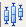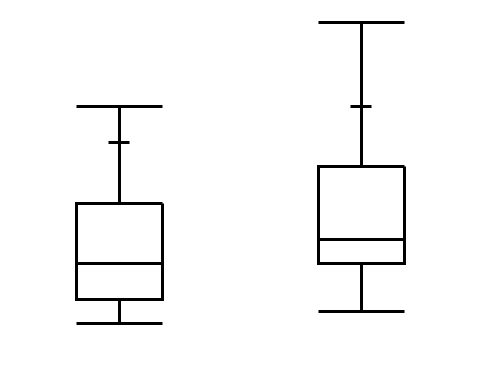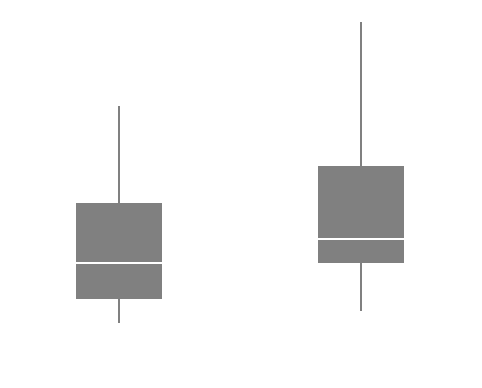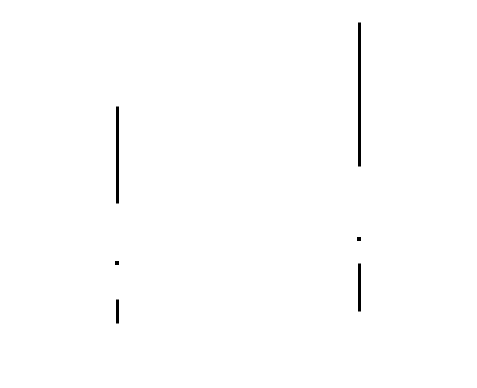The Box Plot elementshows outlier or quantile box plots. A box plot provides a compact view of a distribution of values. The box plot element is useful when variables have a Numeric data type. For details about outlier and quantile box plots, see Outlier Box Plot and Quantile Box Plot in the Basic Analysis book.
 • If one of the variables is continuous and the other is nominal or ordinal, box plots are constructed for values of the continuous variable. There is a box plot for each level of the categorical variable.
 • If both variables are continuous, box plots are constructed for values of the continuous variable on the axis specified as the Response Axis. There is a box plot for each level of the other continuous variable.
Figure 2.33 Box Plot Options
 – Show or hide the elements corresponding to a variable in a zone.
 – Add or remove the effect of applying the Color, Size, Shape, or Freq variable to the variable in the zone.
Tip: If you have multiple graphs, you can color or size each graph by different variables. Drag a second variable to the Color or Size zone, and drop it in a corner. In the Variables option, select the specific color or size variable to apply to each graph.
 Box Style Description Normal Shows the box defined by the quartiles as hollow with only a line at the median.Solid Shows the box defined by the quartiles with solid fill. The line at the median is shown by white space.Thin The box defined by the quartiles is not shown. It is discernible due to the whiskers. The median is denoted by a point.Help created on 7/12/2018